Si5351A

Controlling the Si5351A from an Arduino might at first glance seem rather complex or perhaps even scary. Therefore you might revert to using the RFzero code as it is. But with a little help from your friends from RFzero, you will be in a much better position to tweak the examples, add functionality, or perhaps even write your own formulas and functions to calculate the registers and control the frequency or quadrature I/Q phase shift of the Si5351A.

Theory behind the Si5351A

The Si5351A consists of two synthesizers, three output dividers, one per output channel, and one output stage per channel that are all controlled via the I2C bus. A common oscillator circuit, operating at either 25 MHz or 27 MHz, is also built in, but it needs an external crystal.The synthesizer

If you are not familiar with RF synthesizers, and how they work, you can see them as RF generators, that generate frequencies derived from another frequency, i.e. the reference frequency. In the RFzero this is what the 27 MHz crystal is used for.

A synthesizer consists of three main electronic circuits: a phase comparator, a VCO and a feedback divider. Skyworks names the feedback divider the Feedback Multisynth Divider (FMD). For each different programmed setting of the FMD the VCO will generate a different frequency.

In many RF applications this is all that is needed, if the wanted output frequencies are in wide steps, e.g. in 25 kHz steps used for FM channels.

The output multisynth divider

To improve the frequency resolution, from wide steps to finer steps, the synthesizer in the Si5351A is followed by a divider circuit, which Skyworks names the Output Multisynth Divider (OMD). In the Si5351A Skyworks has added a second divider stage, called R, that can divide the frequency generated by the synthesizer even further.

The drawback of an output divider is that the output frequency (fout) will be lower than the VCO frequency (fvco). In the Si5351A there is a minimum division of four. This means that the VCO frequency is always at least four times higher than the output frequency. But instead of having wide frequency steps, e.g. 25 kHz, the steps can be much lower, e.g. 1 Hz. It all depends on how the FMD and OMD ratios are setup.

The math

If you are still with us please stay a bit longer, and we will take you by the hand and walk you through the math, and how to generate the wanted output frequency (fout) derived from the reference frequency (fref). In math lingo the output frequency can be expressed with the following formula: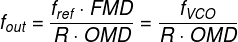The Si5351A FMD and OMD ratios can also be expressed as: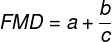Please note that Skyworks also names the OMD variables, a, b and c, but, they are NOT THE SAME as those for the FMD. This is an obvious place to get the math wrong. So to avoid any possible confusion in the RFzero world they are named d, e and f respectively instead. Please remember this if you dive into the RFzero code or Si5351A programming guide. But now we can write the complete relationship between the reference frequency and the output frequency: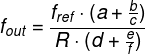That’s easy – right? Hmmm, not quite. We now have one equation with seven variables to get from the reference frequency to the output frequency. But the complexity doesn’t stop here. There are some constraints to the different variables and the electronic circuit too:

• The VCO frequency can only be from 600 MHz to 900 MHz. Yes, over-clocking is possible, and so in under-clocking, to extend the output frequency range beyond 2,3 kHz to 200 MHz. But let’s leave this for now.
• The FMD ratio can be from 15 + 0/1 048 575 and to 90 + 0/1 048 575, i.e. a = 15, b = 0 and c = 1 048 575 to a = 90, b = 0 and c = 1 048 575. Please remember that c can never be zero (0), as this will violate fundamental math principles.
• The OMD ratio can be 4 and from 6 to 2048. Please remember that f can never be zero (0), as this will violate fundamental math principles.
• The R divider can only be 1, 2, 4, 8, 16, 32, 64 or 128.
• If the output frequency is above 150 MHz d is always 4.
• If the output frequency is below 500 kHz R should be used, i.e. higher than 1.

You also need to understand a bit more about synthesizer and divider circuits. The FMD and OMD ratios are what is called fractional, e.g. 10 + 23 / 4567. But synthesizer and divider circuits produce more spurious and phase noise/time jitter when operated in fractional mode than in integer mode, e.g. 10 + 0 / 4567 = 10. So a clever RF designer will assess the situation, and decide if fractional modes are need for both the FMD and the OMD, a mix or use both in integer mode. If a mix is sufficient the OMD should be operated in integer mode. Furthermore, are even numbers for a and d preferred again due to spurious and noise/jitter. The software will have take all this into account.

Agreed, this doesn’t help a lot. But here comes the first trick to get started. Do a calculation using an initial VCO frequency of 600 MHz, if the wanted output frequency is below 150 MHz. So let’s continue with these design advises and choose an output frequency of 28,567 MHz which means that R = 1.

The second trick is then to work backwards from the output frequency by first calculating the R · OMD ratio:This means that d is 21, because R is 1, and the e/f ratio is 0,0032555046032…

This is neither an integer nor an even one if rounded. So the next trick is to round d up to an even integer, i.e. 22, and check if the resulting VCO frequency is still within the valid range from 600 MHz to 900 MHz. If not then increase, or decrease, the value of d by two. Since we want to use the OMD in integer mode, the e/f ratio doesn’t matter, and the FMD will have to take care of this instead. So 22 · 28,567 MHz = 628,474 MHz which is a fine fvco.

We now know that d = 22, e = 0 and f = 1 or any other value as long as f is less than or equal to 1 048 575. So we can continue to find the FMD, i.e. a, b and c:where a = 23 is the integer part of the FMD and b/c = 0,2768148148148… is the remainder.

Hang in there we are almost done. But how do we solve the b/c = 0,2768148148148… equation? The easiest way to do it is to set c to 1 048 575. This results in b equals 290 261.

That’s it! We now have the following values:

• a = 23
• b = 290 261
• c = 1 048 575
• d = 22
• e = 0 (static)
• f = 1 (static)
• R = 1

But will the output frequency be correct then? Well, let’s find out:OK, not spot on but -111 mHz away.

What if the output frequency is less than 500 kHz? In this case the R divider comes into play. Fortunately the procedure is simple. Multiply the wanted output frequency below 500 kHz with a valid R value (1, 2, 4, 8 … 128) so the “new frequency” becomes greater than 500 kHz. Then do the above math using this new frequency as fout in the above equations, e.g. if you want 100 kHz an R = 8 will bring fout up to 800 kHz. The datasheet says that the R divider can be used below 500 kHz, but to get to the lowest frequency the Si5351A can generate, this border frequency should be set to 292 969 Hz instead coming from fvco / dmax = 600 MHz / 2048.

Frequency code for the Si5351A

Below is a function that calculates the FMD, OMD and R variables and returns the register values for the Si5351A. In this code the OMD is set to operate in integer mode, thus e = 0 and f = 1.

The code works for frequencies from 2289 Hz and up. According to Skyworks the Si5351A can work up to 200 MHz. It is actually possible to use the Si5351A beyond 290 MHz, but the maximum frequency depends very much of the actual Si5351A device. Once you get closer to the frequency limit of the Si5351A, you will experience, that the synthesizer has difficulties getting into a steady state (locked). Beyond 200 MHz the signal-to-noise ratio also decreases. Another way to use the Si5351A at higher frequencies is to use one of the harmonics. But please keep in mind that higher output frequency also means worse spectral performance.

Here is an online tool to calculate the FMD, OMD and R register values.

Putting it all together

We are now almost ready generate a frequency with the Si5351A. But before we can do this a pair of I2C (Wire) read and write functions are needed.

The I2C (Wire) write function.

For the RFzero the Wire instance must be replaced by WireLocal.

Initializing the Si5351A

In the below example the structure of the initialization of the Si5351A is taken from the flowchart in the datasheet.

The FMD for PLLA is used and connected to CLK0. The OMD for CLK0 is set to integer mode. Only CLK0 is initialized and with an output stage current of 8 mA. The reference load is set to 6 pF.

The program

The final program is now ready. Add each of the above functions to the .ino file and complete the setup() function.

The setup() and loop() part of the program.

Quadrature I/Q and 90° phase offset

Before diving into the math please remember that two different frequencies can never have a static phase difference. Only the same frequency may have a static phase difference.

In the Si5351A programming guide the below formula for applying a phase offset has to be used:Making a 90° phase offset, e.g. for I/Q quadrature purposes, is very easy when the OMD is operated in integer mode. But first we must find the relationship between toff and 90°. In general it applies that time and frequency are related as:e.g. a signal with a frequency of 1 kHz has a time cycle of 1 ms. Furthermore do we know that one cycle of a signal is 360° thus 90° is one quarter of the cycle: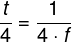From the frequency math we know that the relationship between the wanted frequency and VCO frequency is: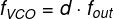Thus we can now put all the elements into the formula: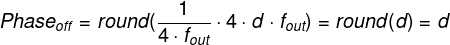So to apply a 90° phase offset load the relevant register with the value of the OMD. Only seven bits are available thus, the max value is 127. However, to keep the OMD an even integer the max value is then 126. Since the specified lower VCO frequency is 600 MHz, the lowest I/Q output frequency is then 4,762 MHz. For a lower frequency please see how to extended the frequency range in the description below.

Extended frequency range

As mentioned in the theory section it is possible to extend the frequency range by “overclocking” or “underclocking” the Si5351A. This means that the VCO will operate over or under its specified frequency range from 600 MHz to 900 MHz. In either case the outer limits are very device specific. This means that one Si5351A may go a bit further than another one, and you cannot tell before trying.

When operating outside the specified frequency range, from 600 MHz to 900 MHz, the RF performance is reduced which means worse spectrum. When operating close to the limits, but still in lock, the signal becomes much less clean. When operating outside the min/max VCO frequency range, the PLL will not lock, thus the signal becomes very unstable and often useless.

Empirical data shows that the typical maximum VCO frequency is slightly lower than 1,2 GHz. Given that the minimum OMD is 4, this means that the maximum output frequency is somewhere between 290 MHz and 300 MHz. At the lower end the minimum VCO frequency is around 420 MHz resulting in a minimum output frequency around 2 kHz. For I/Q purposes the minimum output frequency is around 3,3 MHz with an even integer OMD.